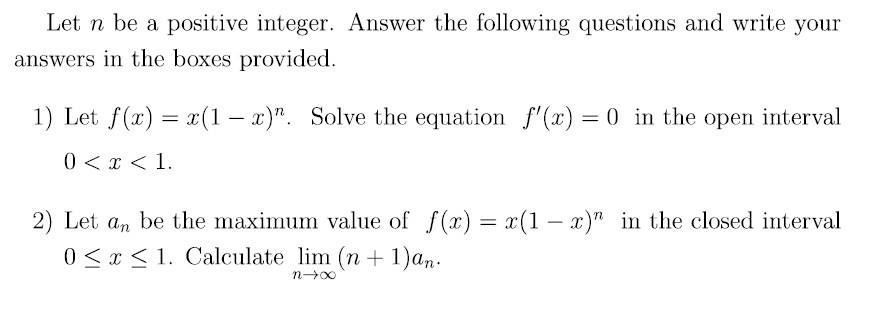# Differentiate f(x)=x(1-x)^n

Homework Statement:
help with my work
Relevant Equations:
derivative and integral
Hello, good afternoon guys. I need a little help from you: D

1)n a positive integer.
f(x)=x(1-x)^n
Solve the equation f'(x)=0 in 0<x<1

this question I asked and found x = 1 / (x + 1) ok

2)An be the maximum value of f(x)=x(1-x)^n in [0,1]
Calculate lim (n+1)an
n tending to infinity

the maximum value would not be making the derivative equal to zero? would it be x = 1? I did not understandLast edited by a moderator:

nrqed
Homework Helper
Gold Member
Homework Statement:: help with my work
Relevant Equations:: derivative and integral

Hello, good afternoon guys. I need a little help from you: D

1)n a positive integer.
f(x)=x(1-x)^n
Solve the equation f'(x)=0 in 0<x<1

this question I asked and found x = 1 / (x + 1) ok

2)An be the maximum value of f(x)=x(1-x)^n in [0,1]
Calculate lim (n+1)an
n tending to infinity

the maximum value would not be making the derivative equal to zero? would it be x = 1? I did not understand View attachment 264068
You did not do the first part correctly.

What is the derivative f'(x)?

•Delta2 and member 587159
osilmag
Gold Member
Help, you could use the product rule, then set it equal to zero.

vela
Staff Emeritus